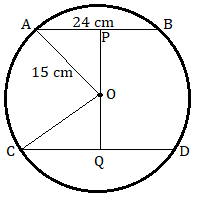Request a call back

Two parallel chords are drawn in a circle of diameter 300 cm. The length of one chord is 24.0 cm and the distance between the two chords is 21.0 cm; find the length of the other chord.
Asked by siddeshmankar90.9spicertl | 06 Feb, 2021, 02:16: PMExpert AnswerLet AB = 24 cm be the first chord and CD be the second chord in the circle with centre O.
P and Q are on AB and CD bisecting them respectively.
So, AP = PB = 12 cm
In triangle APO, by Pythagoras theorem we have
PO2 = AO2 - AP2 = 152 - 122 = 81
Therefore, PO = 9 cm
So, we get OQ = 21 - 9 = 12 cm
In triangle COQ, by Pythagoras theorem we have
CQ2 = CO2 - OQ2 = 152 - 122 = 81
Therefore, CQ = 9 cm
Thus, CD = 2CQ = 18 cm
Answered by Renu Varma | 09 Feb, 2021, 12:55: PM

## Application Videos

ICSE 9 - Maths
Asked by siddeshmankar90.9spicertl | 06 Feb, 2021, 02:16: PMANSWERED BY EXPERT
ICSE 9 - Maths
Asked by siddeshmankar90.9spicertl | 06 Feb, 2021, 01:57: PMANSWERED BY EXPERT
ICSE 9 - Maths
Asked by sharmasukhjinder7 | 10 Jan, 2020, 11:55: AMANSWERED BY EXPERT
ICSE 9 - Maths
Asked by Sumitavbeyond | 29 Jan, 2018, 06:17: PMANSWERED BY EXPERT
ICSE 9 - Maths
Asked by pereiracalida | 17 Jan, 2018, 11:00: PMANSWERED BY EXPERT
ICSE 9 - Maths
Asked by pereiracalida | 17 Jan, 2018, 10:47: PMANSWERED BY EXPERT
ICSE 9 - Maths
Asked by pereiracalida | 17 Jan, 2018, 10:33: PMANSWERED BY EXPERT
ICSE 9 - Maths
Asked by pereiracalida | 14 Jan, 2018, 07:36: PMANSWERED BY EXPERT
ICSE 9 - Maths
Asked by pereiracalida | 14 Jan, 2018, 07:29: PMANSWERED BY EXPERT
ICSE 9 - Maths
Asked by pereiracalida | 14 Jan, 2018, 07:26: PMANSWERED BY EXPERT